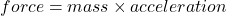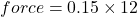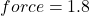## What is the net force acting on a .15 kg hockey puck accelerating at a rate of 12 m/s2

Question

What is the net force acting on a .15 kg hockey puck accelerating at a rate of 12 m/s2

in progress 0
4 months 2021-09-05T15:28:50+00:00 2 Answers 8 views 0

The net force is 1.8N

Explanation:

Given that the formula for force is Force = mass×acceleration. So you have to substitute the values into the formula :Let mass = 0.15kg,

Let acceleration = 12m/s²,1.8 Newtons

Explanation:

We want to figure out the net force acting on a 0.15 kg hockey puck that has an acceleration of 12 m/s². To solve this, we must apply Newton’s Second Law of Motion which is denoted by the formula: f = ma.

Here, f is the force, m is the mass, and a is the acceleration. Plug our given values in to find f:

f = ma

f = (0.15 kg) * (12 m/s²) = 1.8 Newtons

Thus, the answer is 1.8 N.

~ an aesthetics lover